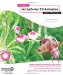# Easing and SpringingEasing and springing are also not much more complex in 3D than they are in 2D (the subject of Chapter 8). You mainly need to add in another variable or two for the z axis, and youre all set.

### Easing

Theres not a whole lot to cover on easing. In 2D, you have tx and ty as a target point. You just add tz for the z axis. On each frame, you measure the distance from the object to the target on each axis, and move it a fraction of the way there.

Lets look at a simple example that eases an object to a random target, and when it gets there, it picks another target and moves the object there. Note that I went back to my red ball again for the next couple of examples. Heres the code ( ch15_09.fla ):

` var x:Number = 0; var y:Number = 0; var z:Number = 0; var tx:Number = Math.random() * 500 - 250; var ty:Number = Math.random() * 500 - 250; var tz:Number = Math.random() * 500; var easing:Number = .1; var fl:Number = 250; var vpX:Number = Stage.width / 2; var vpY:Number = Stage.height / 2; function onEnterFrame():Void {       var dx:Number = tx - x;       var dy:Number = ty - y;       var dz:Number = tz - z;       x += dx * easing;       y += dy * easing;       z += dz * easing;       var dist:Number = Math.sqrt(dx*dx + dy*dy + dz*dz);       if(dist < 1)       {             tx = Math.random() * 500 - 250;             ty = Math.random() * 500 - 250;             tz = Math.random() * 500       }       if(z <= -fl)       {             ball._visible = false;       }       else       {             ball._visible = true;             var scale:Number = fl / (fl + z);             ball._xscale = ball._yscale = scale * 100;             ball._x = vpX + x * scale;             ball._y = vpY + y * scale;       } } `

The most interesting point of this code is the following line:

` var dist:Number = Math.sqrt(dx*dx + dy*dy + dz*dz); `

If you remember, in 2D, you measure the distance between two points by the following equation:

` var dist:Number = Math.sqrt(dx*dx + dy*dy); `

To move into 3D distances, just add the square of the distance on the third axis. This always strikes me as too simple. It seems like I should be using a cube root instead of a square root, now that Ive added on an extra term . But it doesnt work that way.

### Springing

Springing, being a close cousin to easing, requires a similar adjustment for 3D. You just use the distance to the target to change the velocity, rather than the position. Ill give you another quick example. In this one (file ch15_10.fla ), clicking the mouse will create a new random target for the ball to spring to.

` var x:Number = 0; var y:Number = 0; var z:Number = 0; var vx:Number = 0; var vy:Number = 0; var vz:Number = 0; var tx:Number = Math.random() * 500 - 250; var ty:Number = Math.random() * 500 - 250; var tz:Number = Math.random() * 500; var friction:Number = .98; var spring:Number = .1; var fl:Number = 250; var vpX:Number = Stage.width / 2; var vpY:Number = Stage.height / 2; function onMouseDown():Void {       tx = Math.random() * 500 - 250;       ty = Math.random() * 500 - 250;       tz = Math.random() * 500; } function onEnterFrame():Void {       var dx:Number = tx - x;       var dy:Number = ty - y;       var dz:Number = tz - z;       vx += dx * spring;       vy += dy * spring;       vz += dz * spring;       x += vx;       y += vy;       z += vz;       vx *= friction;       vy *= friction;       vz *= friction;       if(z <= -fl)       {             ball._visible = false;       }       else       {             ball._visible = true;             var scale:Number = fl / (fl + z);             ball._xscale = ball._yscale = scale * 100;             ball._x = vpX + x * scale;             ball._y = vpY + y * scale;       } } `

As you can see, this uses the basic spring formula (from Chapter 8) with a third axis.Foundation Actionscript 3.0 Animation: Making Things Move!
ISBN: 1590597915
EAN: 2147483647
Year: 2005
Pages: 137
Authors: Keith Peters

Similar book on Amazon